PSAT Math : Permutation / Combination

Example Questions

2 Next →

Example Question #11 : How To Find The Greatest Or Least Number Of Combinations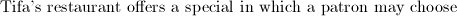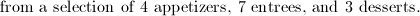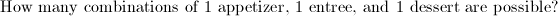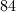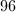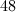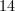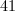Explanation: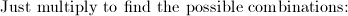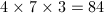Example Question #11 : How To Find The Greatest Or Least Number Of Combinations

A group of friends decide to go out to the movies.  Fred and Tom are bringing dates, while their 2 friends are going alone.  When the friends arrive at the movie theater, they find a row of six seats so they can all sit together.

If Fred and Tom must sit next to their dates, how many different ways can the group sit down?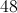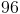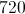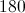Explanation:

Think of the seats as an arrangement of people in a line.  Fred and Tom must sit next to their dates, so you can treat the pair as a single object.  The only difference is that we must then multiply by 2, since we can switch the order in which they sit down at will (either Fred or his date can sit on the left).

So instead of dealing with 6 objects, we now simply work with 4.  An arrangement of 4 objects, can be made in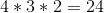different ways.  You can choose any of 4 objects to be in the first spot. Once that spot is taken, you move onto the next of four spots.  You place any of the remaining three there, giving you 3 more choices (or multiplying by 3).  You do the same thing 2 more times to end up with 24 possibilities.

Finally, you have to take into account switching the positions of Fred/Tom and their respective dates.  Since there are two pairs, you multiply by 2 twice.  This gives you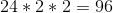different arrangements.

Example Question #11 : Permutation / Combination

There are seven unique placemats around a circular table. How many different orders of placemats are possible?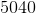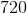Explanation:

Since the table is circular, you need to find the total number of orders and divide this number by 7.

The total number of different orders that the placemats could be set in is 7! (7 factorial).

7!/7 = 6! = 720

Note that had this been a linear, and not circular, arrangement there would be no need to divide by 7. But in a circular arrangement there are no "ends" so you must divide by N! by N to account for the circular arrangement.

Example Question #11 : How To Find The Greatest Or Least Number Of Combinations

For a certain lunch special, customers must order a salad, an entree, and a dessert. If there are three different salads, four different entrees, and two different desserts available, then how many different lunch specials are possible?

12

72

18

24

9

24

Explanation:

Customers must choose a salad, an entree, and a dessert. There are three different salads, four entrees, and two desserts.

The simplest way of determining the number of combinations is by multiplying the number of options for each part of the meal. In other words, we can find the product of 3, 4, and 2, which would give us 24.

Sometimes, if you can't think of a way to mathetimatically determine all of the different combinations of something, it helps to write out as many as you can. Let's write out all of the possible cominbations just to verify that there are 24. Let's call the different salads S1, S2, and S3. We will call the four entrees E1, E2, E3, and E4, and we will call the desserts D1 and D2.

Here are the possible lunch special combinations:

S1, E1, D1

S1, E1, D2

S1, E2, D1

S1, E2, D2

S1, E3, D1

S1, E3, D2

S1, E4, D1

S1, E4, D2

S2, E1, D1

S2, E1, D2

S2, E2, D1

S2, E2, D2

S2, E3, D1

S2, E3, D2

S2, E4, D1

S2, E4, D2

S3, E1, D1

S3, E1, D2

S3, E2, D1

S3, E2, D2

S3, E3, D1

S3, E3, D2

S3, E4, D1

S3, E4, D2

Example Question #11 : Permutation / Combination

There are five pictures but only four display cases.  The display cases are unique. How many different arrangements of pictures in display cases can be created?

5

50

240

24

120

120

Explanation:

There are five possible choices for the first space. For the second there are four possible, three for the third, and two for the fourth. 5 * 4 * 3 * 2 = 120 possible arrangements.

Example Question #1891 : Psat Mathematics

The art club must choose a leadership committee of 3 students.  If any

member can be on the committee, how many different combnations of 3

students can be selected from the 15 members of the art club?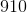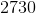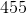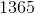Explanation: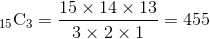Example Question #1 : How To Find Permutation Notation

A company assigns employee numbers according to the following scheme:

1) Each number must comprise three letters, followed by five numerals.

2) The first numeral must be either '1' or '2'; there are no other restrictions on the numerals.

3) There cannot be repetition among the three letters.

4) To prevent confusion with the numerals '1' and '0', the letters 'I' and 'O' cannot appear.

Which of the following expressions is equal to the number of possible employee numbers?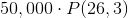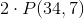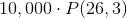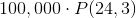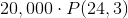Explanation:

The first three characters must be distinct letters, none of which are 'I' or 'O', meaning that three letters will be selected from a set of 24. Also, order will be important, so the number of ways to choose this group of three will be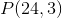.

The letters are followed by either a '1' or a '2', so this makes 2 possibilities.

The next three characters will be numerals, and there will be no restrictions, so the number of ways to choose this group will be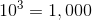.

By the multiplication principle, the number of ways to choose an employee number will be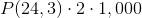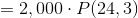Example Question #11 : Permutation / Combination

A company assigns employee numbers according to the following scheme:

1) Each number must comprise three letters, followed by four numerals, followed by one letter.

2) There are no restrictions on the numerals.

3) There cannot be repetition among the first three letters; however, the final letter can be any letter, even if that letter is among the first three.

Which of the following expressions is equal to the number of possible employee numbers?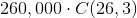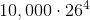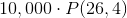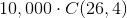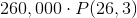Explanation:

The first three characters must be distinct letters, meaning that three letters will be selected from a set of 26. Also, order will be important, so the number of ways to choose this group of three will be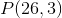.

The next four characters will be numerals, with no restrictions, so the number of ways to choose this group will be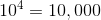The last character can be any of 26 letters.

By the multiplication principle, the number of ways to choose an employee number will be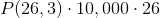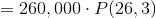Example Question #11 : Permutation / Combination

A company assigns employee numbers according to the following scheme:

1) Each number must comprise two letters, followed by four numerals, followed by two letters.

2) There are no restrictions on the numerals.

3) There cannot be repetition between the first two letters.

4) There cannot be repetition between the last two letters.

5) A letter appearing as one of the first two letters can appear as one of the last two.

Which of the following expressions is equal to the number of possible employee numbers?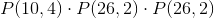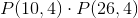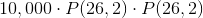Explanation:

The first two characters must be distinct letters, meaning that two letters will be selected from a set of 26. Also, order will be important, so the number of ways to choose this group of two will be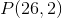.

Similarly, since the last two characters will be chosen according to the same rule, with repetition allowed between the two groups, there will beways to choose them as well.

The next four characters will be numerals, but there will be no restrictions, so the number of ways to choose this group will be.

By the multiplication principle, the number of ways to choose an employee number will be2 Next →